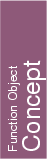## Kernel::LessSignedDistanceToPlane_3

A model for this must provide:

bool
 fo.operator() ( Kernel::Plane_3 p, Kernel::Point_3 q, Kernel::Point_3 r)
returns true, iff the signed distance from point q to plane p is smaller than the signed distance from point r to p.

bool
 fo.operator() ( Kernel::Point_3 p1, Kernel::Point_3 p2, Kernel::Point_3 p3, Kernel::Point_3 q, Kernel::Point_3 r)
returns true, iff the signed distance from point q to the plane p defined by p1, p2, p3 is smaller than the signed distance from point r to p.
Precondition: p, q, and r are not collinear.